/

### Function Description

Returns the size of a confidence interval for the mean of a sample, using a Student’s t distribution, i.e. returns: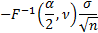where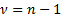,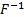is the inverse cumulative distribution function for the Student’s t distribution with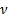degrees of freedom,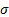is the standard deviation andis the sample size.

Provided for compatibility with the CONFIDENCE.T function in Microsoft Excel and some other spreadsheet packages. Please note that the Nematrian function accepts non-integral values of(these can arise if observations are given different weights).

Contents | Prev | Next